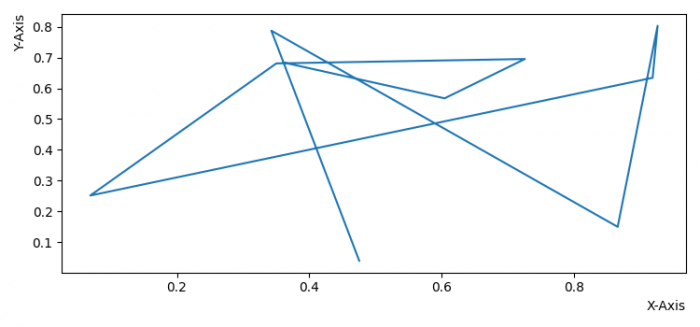# How to align axis label to the right or top in Matplotlib?

MatplotlibPythonData Visualization

To align axis label to the right (X-axis label) or top (Y-axis label), we can take the following steps −

• Set the figure size and adjust the padding between and around the subplots.
• Create a figure and a set of subplots.
• Initialize a variable, N, for number data samples.
• Plot x and y data points using plot() method.
• Set xlabel and ylabel at the right and top locations, respectively.
• To display the figure, use show() method.

## Example

import numpy as np
from matplotlib import pyplot as plt

plt.rcParams["figure.figsize"] = [7.50, 3.50]
plt.rcParams["figure.autolayout"] = True

fig, ax = plt.subplots()
N = 10
x = np.random.rand(N)
y = np.random.rand(N)

ax.plot(x, y)
ax.set_xlabel("X-axis", loc="right")
ax.set_ylabel("Y-axis", loc="top")

plt.show()

## Output